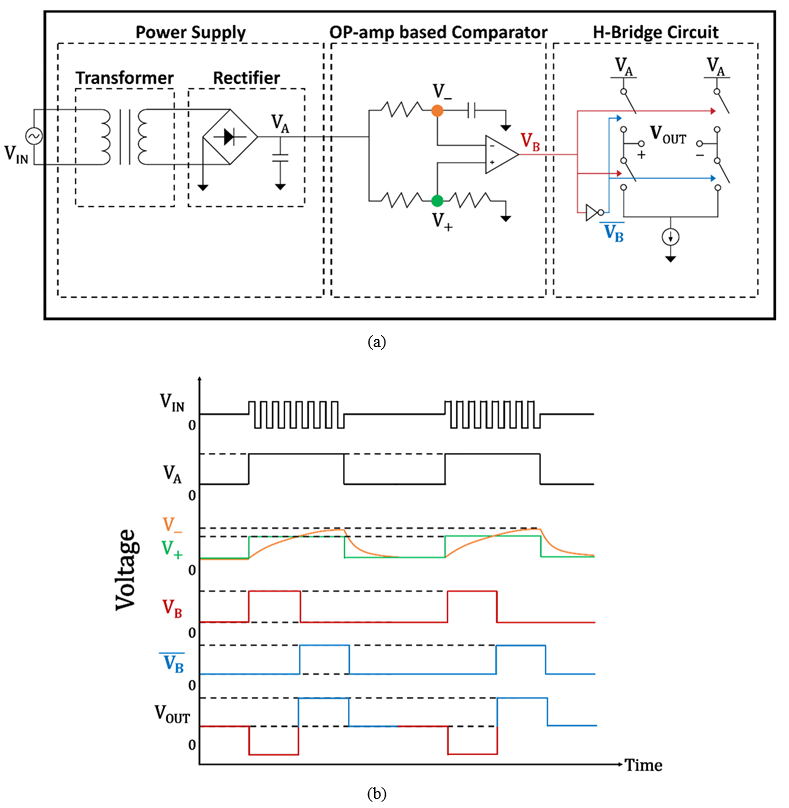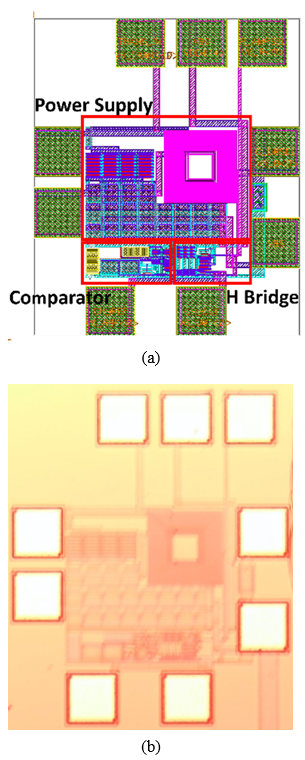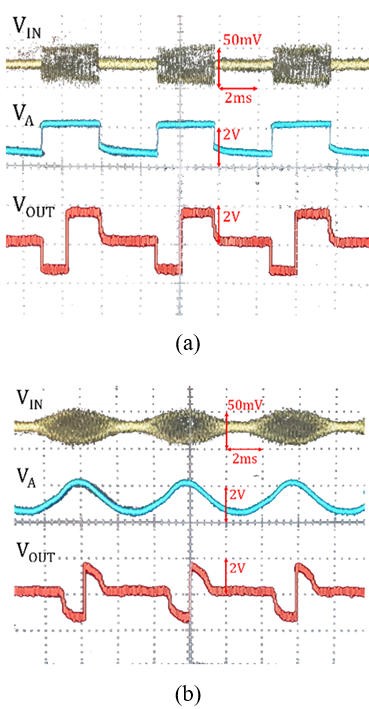Mobile QR Code1. (Department of Electrical and Computer Engineering, College of Engineering, Seoul National University, Seoul 08826, Korea)
2. (Brain Science Institute, Korea Institute of Science and Technology, Seoul 02792, Korea)
3. (Pratt School of Engineering, Duke University, Durham 27708, U.S.A. (On a leave of absence) )

Biphasic pulses, signal generator, demodulation, neural prosthesis, potentiostat

## I. INTRODUCTION

Electroanalytical systems have included electrical signal generators, as generated signals with sinusoidal or non-sinusoidal waveforms are used as references for electroanalytical experiments and output responses generated by sensors and transducers.

A potentiostat with a two or three-electrode setup is one of the most representative applications which show how these signals are used in electroanalytical experiments (1). This device enables a variety of electroanalytical methods ranging from linear sweep voltammetry (LSV) to electrochemical impendence spectroscopy (EIS) and cyclic voltammetry (CV). Each method requires dynamic controls in cycling the potential on working electrodes. For example, cyclic voltammetry is a technique to plot cyclic voltammogram which shows the reaction progress of the chemical systems by supplying the repeating scan cycles of sawtooth voltage waves on working electrodes.

Electrochemical devices and workstations such as a potentiostat have been miniaturized as they began to be used in nanoscale semiconductor/integrated circuit failure analysis and implantable neural prosthetics such as neural recordings, cochlear implants, and deep brain stimulation (DBS) for patients with neurological disorders. For example, a quantum-mechanical system is used to reveal the characteristics of defects in semiconductor materials with nanometer resolution (2). The system used the excitonic transition of the quantum dot to introduce the quantum sensors for nanoscale defect characterization. Moreover, in vivo neural recording and neuromodulation techniques have led to the developments of many miniaturized electroanalytical devices which monitor in vivo neuro-electrical activities and generate bioelectrical signals by achieving wireless data telemetry and biocompatible packaging (3). A portable wireless instantaneous neuro transmitter concentration sensing (WINCS) system is one of the examples which applied Fast Scan Cyclic Voltammetry (FSCV) to enable in vivo high-resolution dopamine detections with data telemetry (4).

As miniaturized electroanalytical systems have made inroads into applications in nanoscale system analysis and implantable neural prosthetics, the needs for signal generators integrated with minimal circuits surrounding the IC have been also increasing. Here, we suggest an integrated circuit which generates biphasic pulses and changes their parameters depending on the parameters of the amplitude modulated (AM) input with a 1Ghz carrier. This design allowed the system to generate various pulses without additional surrounding interfaces, and it only required a single external tuning capacitor to achieve desired waveforms more precisely. We examined the performances of the system to prove that this system could implement dynamic controls in potential just by using the changes of the supply input. If the parameters of generated biphasic pulses vary on a chip without any additional interfaces, the whole size of a multi-channel biphasic pulse generator system can be miniaturized to achieve distributed neural interfaces with a higher resolution. In addition, this system also included a miniaturized transformer on the integrated circuit and showed that an integrated transformer stepped up the input with a 1 Ghz carrier, which suggests an integrated circuit for a wireless electroanalytical system on a chip as a future work.

## II. CIRCUIT DESCRIPTION

Design of the biphasic pulse generator IC is explained with the help of block and timing diagrams in Fig. 1. In the IC, there were functional blocks of a Power Supply, an OP-amp based Comparator, and an H-bridge circuit. The Power Supply block consisted of a step-up transformer and a full-wave rectifier. These are schematically depicted in Fig. 1(a) with timing diagrams of important node voltages shown in Fig. 1(b).

The source signal (V$_{IN}$) was an amplitude-modulated (AM) signal with a radio frequency carrier. While it was supplied directly within the scope of current work, it can also be wirelessly transmitted if a separate receiving coil is provided. The carrier frequency was 1GHz so that the prospective receiving coil be of a size that can be monolithically integrated.

V$_{IN}$ was stepped up, in terms of voltage, after passing through the transformer. Transformer was in an interleaved design, where the primary coil and the secondary coil are on the same metal layer.

The full-wave rectifier circuit was made of four MOS diodes and a capacitor. It detected an envelope of the stepped-up voltage (V$_{A}$), which was then fed to two places. First, it was connected to the comparator stage as the input to the OP-amp. Secondly, V$_{A}$ provided supply voltage for the remaining circuits including the H-bridge circuit.

The OP-amp worked as a comparator, and this was where the monophasic pulse in V$_{A}$ became biphasic. To generate biphasic pulse, the output change of the OP-amp from High to Low must occur once. An inverting input of the OP-AMP (V$_{-}$) was connected to the node between a resistor and a capacitor, which causes an RC time delay on the voltage of the inverting input (V$_{-}$). Whereas the inverting input of the OP-amp (V$_{-}$) was connected to an RC circuit, a non-inverting input of the OP-amp (V$_{+}$) was connected to a voltage-divider circuit. A divided voltage on the non-inverting input (V$_{+}$) worked as a reference to be compared with that on the inverting input (V$_{-}$).

The operation in the comparator can be further illustrated using timing diagrams of corresponding node voltages shown in Fig. 1(b). For the initial phase of the pulse in V$_{A}$, the voltage on the non-inverting input of the OP-amp (V$_{+}$) was higher than that on the inverting input (V$_{-}$). Therefore, the output voltage of the OP-amp (V$_{B}$) was High at first. However, the voltage on the inverting input (V$_{+}$) increases over a certain period of time, and its value becomes higher than that of the non-inverting input (V$_{+}$). At that moment, the output voltage of the OP-amp (V$_{B}$) switches from High to Low.

This shift allowed the H-bridge circuit to provide the switched polarity of an output voltage, which resulted in a biphasic pulse. The elapsed time which it took for the voltage on the inverting input (V$_{-}$) to rise above that on the non-inverting input (V$_{+}$) was the duration of a cathodic phase in the biphasic pulse. A duration of an anodic phase of the biphasic pulse was the elapsed time from the moment which the voltage on the inverting input(V$_{+}$) was higher, until the end of the pulse duration in V$_{A}$.

Fig. 1. (a) The system block diagram for the designed IC with (b) key node voltages showing how the system works.The duration of the monophasic pulse in V$_{A}$ was now divided into two parts in the biphasic V$_{B}$. The timing of the division could be adjusted by adjusting the RC time. In the current work, an external tuning chip capacitor was used for the adjustment.

While control of the switches in an H-bridge was commonly done with digital programming (5), we took an analog approach to control the switches. The operation of the H-bridge circuit is explained using the blocks in Fig. 1(a) and the timing diagrams in Fig. 1(b).

While V$_{B}$ was used to control a pair of pull-up and pull-down transistors (colored in red), its inversion ($\overline{V_{B}}$) controls the other pair (colored in blue). The direction of the current that flows between the two output electrodes of the H-bridge circuit, reverses at the time the two phases cross. This action generated the desired biphasic current pulses flowing through the output nodes. Depending on which pair of the transistor switches are enabled first, the resulting biphasic pulse can be either negative-first or positive-first.

The space between the two output electrodes may be filled with an electrolyte, and we may assign an impedance to represent the space. Then the biphasic current pulse flowing through the impedance becomes a biphasic voltage. This can be seen in the color traces of V$_{OUT}$ in Fig. 1(b) where colors match those used in the circuit of Fig. 1(a).

The duration and rate of the biphasic pulse (V$_{OUT}$) were the same as the ones given in the input signal (V$_{IN}$). The switching time between the negative and the positive phases of the pulse was determined by the tuning capacitor connected to the inverting input (V$_{-}$) of the OP-amp, and by a reference voltage set by two on-chip resistors connected to the non-inverting input node (V$_{+}$) of the OP-amp. The parameters of pulse such as amplitude, frequency, and duration of the biphasic output (V$_{OUT}$) were determined by those in the supply voltage of the H-bridge (V$_{B}$) which, in turn, depended on those of the rectified envelope signal V$_{A}$ and of the input signal V$_{IN}$.

## III. MEASUREMENT RESULTS

Fig. 2. (a) A layout of the whole integrated circuit. Its size is 441.5 μm 527.8 μm including bond pads, (b) A microphotography of the fabricated integrated circuit.The designed IC was fabricated by using the MagnaChip/SK Hynix CMOS 0.35μm process. Shown in Fig. 2(a) and (b) are the layout of the designed IC and a microphotography of the fabricated chip, respectively. The size of the fabricated chip was 441.5 μm × 527.8 μm including bond pads.

To test the fabricated IC chip, a square wave source signal (V$_{IN}$) was used, which was amplitude-modulated with a 1 Ghz carrier. A radio frequency signal generator (Agilent ESG-D Series E4433B signal generator) was used to provide the source. The output waveforms were garnered using an oscilloscope (TDS3054B, Tektronix, Inc., U.S.A).

Fig. 3. Typical waveforms measured at key nodes in Fig. 1. Input signals were amplitude modulated with 1 GHz carrier for (a) a square wave, (b) a sinusoidal input, respectively.In Fig. 3(a), typical waveforms are shown. In this case, the amplitude of the pulse in V$_{IN}$ was approximately 50 mV which was a 6 dB output from the Agilent generator. V$_{A}$, the rectified output had pulse amplitude of approximately 1.5 V, and biphasic pulses were observed in the output (V$_{OUT}$) with approximately 2 V amplitude in both negative and positive directions. The biphasic pulses were negative-first as intended. The repetition rate was 170 Hz and an external tuning capacitor of 8.2 nF was used.

The IC produced similar waveforms when a sinusoidal input waveform was used instead of the square one, as shown in Fig. 3(b). When compared with the waveforms shown in Fig. 3(a), one may notice a difference in the envelope of the biphasic output having a sinusoidal shape, as expected for a sinusoidal input.

The minimum voltage of V$_{A}$ at which the biphasic pulse generation occurred was about 1 V and this is due to the fact that V$_{A}$ was used as the supply voltage for the comparator and H-bridge circuits. The IC worked well up to 3.3 V of V$_{A}$, which is the power supply voltage of the IC.

The chip was also tested for various repetition rates. Clear biphasic pulses were generated at the rates of 170, 500, and 1.2 K Hz, for which the off-chip tuning chip capacitors of 8.2, 3.3, and 1.2 nF were used, respectively. When tested at 10 K Hz, however, the waveforms of the supply voltage and the biphasic pulses were distorted.

## IV. ISCUSSIONS AND CONCLUSIONS

A full-custom integrated circuit was designed, fabricated, and tested, that generated biphasic pulses out of monophasic, amplitude-modulated input signals. Adjustments of all three pulse parameters (amplitude, duration, and repetition rate) were made. Similar works have been made by other groups in the past (3,6,7) and results of this work can be compared with the other published works as summarized in Table 1.

Table 1. Comparison of performances of prior researches and this work

    This work Process 130 nm RFCMOS 65 nm LPCMOS - 350 nm CMOS Operation voltage (V) 1.2–1.3 2.5 - >1, <3.3 Die size (μm$^{2}$) 100x200 (excluding pads) 150x400 (including pads) 1100x1100 (including pads) 441.5x527.8 (including pads) Stimulation strategy Monophasic current or voltage pulse Monophasic current pulse Monophasic voltage pulse Biphasic current pulse Stimulation parameter Invariable amplitude, Variable duration and pulse rate according to RF-transferred power signal Variable amplitude, duration, and pulse rate according to ultrasonic power signal Variable amplitude according to RF-transferred power signal, Invariable duration Variable amplitude, duration, and pulse rate according to RF-transferred power signal Wireless power and data transfer On-chip coil with transmission frequencies between 800 MHz and 2 GHz (power transfer) Piezo-crystal for ultrasonic communication (power and control signal transfer) External coil with a resonant frequency of 394 MHz (power transfer) On-chip coil with a transmission frequency of 1 GHz (power transfer)

This work is distinguished from other works in that it has succeeded in generating biphasic pulses while the others have produced monophasic pulses only. We were able to make adjustment to all three relevant pulse parameters (amplitude, duration and rate) as the others have invariable parameters (3,7).

The other teams were successful in transmitting the modulated input signal wirelessly either using on-chip receiving coil (3) or an off-chip one (7). We, on the other hand, were not able to make the wireless transmission work. Even though we had, in a separate chip, a design that incorporated an on-chip receiving coil, the on-chip transformer did not have enough step-up ratio to make the supply voltage (V$_{A}$) surpass the threshold voltage(1V) needed for the node. In the future work, we are planning to incorporate an alternative receiving coil and signal processing circuitry to resolve this issue.

The IC worked for repetition rates up to 1.2 KHz. Further studies on analyzing the characteristics of components used in this IC such as reverse recovery time and parasitic capacitances would be required to make the IC to work at the higher frequency.

In summary we have demonstrated a simplified circuit that can generate biphasic pulses from monophasic input signals where adjustment of pulse parameters was readily made. Even though it needs additional work to complete the wireless link, we think the miniaturized pulse generator described in this work can be applied to achieve a high-resolution analysis on various chemical and electrical systems. In addition, it can allow more efficient distributed neural interfacing system for high-resolution stimulation on a broad area of neurons, while conventional pulse generators for distributed neural interfaces can only generate monophasic and invariable pulses.

### ACKNOWLEDGMENTS

The chip fabrication and EDA tool were supported by the IC Design Education Center (IDEC), Korea. This work was supported in part by a grant by the Samsung Electronics Corp. (#5264-20180116).

### REFERENCES

1
Scholz Fritz, 2014, Electroanalytical Methods Guide to Experiments and Applications, Springer Berlin2
Kerski J., 2021, Quantum Sensor for Nanoscale Defect Characterization, Physical Review Applied, Vol. 15, No. 23
Khalifa Adam, 2018, The Microbead: A Highly Miniaturized Wirelessly Powered Implantable Neural Stimulating System., IEEE transactions on biomedical circuits and systems, Vol. 12, No. 3, pp. 521-5314
Lee Kendall H., 2017, WINCS Harmoni: Closed-Loop Dynamic Neurochemical Control of Therapeutic Interventions, Scientific Reports, Vol. 7, No. 15
An Soon, Jun 2007, Design for a Simplified Cochlear Implant System, IEEE Transactions on Biomedical Engineering, Vol. 54, No. 6, pp. 973-9826
Johnson Benjamin C., 2018, StimDust: A 6.5 mm 3, wireless ultrasonic peripheral nerve stimulator with 82% peak chip efficiency, 2018 IEEE Custom Integrated Circuits Conference (CICC), IEEE, pp. 1-47
Cho Sung, 2012, A SU-8-based fully integrated biocompatible inductively powered wireless neurostimulator, Journal of Microelectromechanical Systems, Vol. 22, No. 1, pp. 170-176## Author

Shinyong Shim received the B.S. and Ph.D. degrees in the Department of Electrical and Computer Engineering from Seoul National University (SNU), Seoul, Korea, in 2015 and 2020, respectively.

He is currently a postdoctoral research associate at the Brain Science Institute, Korea Institute of Science and Technology (KIST), Seoul, Korea, from 2020.

His recent research interests include artificial vision for the blind and system design of ultrasonic imaging as well as ultrasound-based neuromodulation.

Jaehoon Sung is currently an undergraduate student (on a leave) in Pratt School of Engineering at Duke University.

His current intended major is Biomedical Engineering and Electrical & Computer Engineering.

He is interested in bioelectronics, neural prosthetics, neuromodulation, and bioneural prosthetics, and biomechatronics.

Sung June Kim received his B.S. degree in electronics engineering from Seoul National University (SNU) in 1978.

He received M.S. and Ph.D. degrees in electrical engineering from Cornell University, Ithaca, NY, in 1981 and 1983, respectively.

During 1983–1989, he worked as a member of the technical staff with Bell Laboratories, Murray Hill, NJ.

In 1989, he returned to Korea to join SNU, where he is now a Professor Emeritus in electrical and computer engineering.

During 2000–2009, he has served as a director of the Nano-Bioelectronics and System Research Center (NBS).

He has also served as a director of the Interuniversity Semiconductor Research Center (ISRC) during 2010-2012.

His research interest includes neural prostheses for hearing, vision, and motion impaired patients, electrical and optical neural interface, and semiconductor devices.

He is a fellow of IEEE.